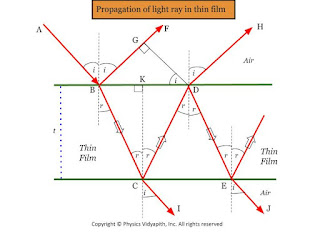### Interference of light due to thin film

Derivation of interference of light due to thin-film: Let's consider a Ray of light $AB$ incident on a thin film of thickness $t$ and the refractive index of a thin film is $\mu$

The ray $AB$ is partially reflected and partially transmitted at $B$. The transmitted BC is against partially transmitted and partially reflected at $C$. The reflected ray $CD$ is partially reflected and partially refracted at $D$.Propagation of light ray in thin film
The interference pattern in reflected light will be due to ray $BF$ and $DH$ which are coherent as they are both derived from the same Ray $AB$.

The interference pattern in transmitted light will be due to ray $CI$ and $EJ$.

The path difference between $BF$ and $DH$ ray will be

$\Delta=\mu(BC+CD)-BG \qquad(1)$

The triangle $\Delta BCK$ and $\Delta CDK$ are congruent because

$\begin{Bmatrix} BK=KD \\ BC=CD \\ CK=t \end{Bmatrix} \qquad (2)$

From equation $(1)$ and $(2)$

$\Delta=2 \mu BC-BG \qquad(3)$

In $\Delta BCK$

$cos \: r= \frac{CK}{BC}$

$BC= \frac{t}{cos \: r} \qquad(4)$

In $\Delta BGD$

$sin \: i= \frac{BG}{BD}$

$BG= BD \: sin\: i$

$BG= (BK+KD)\: sin\: i$

$BG= 2BK \: sin\: i \qquad(5)$ {From eqaution $(2)$}

Again In $\Delta BCK$

$tan \:r = \frac{BK}{CK}$

$BK=t.tan\:r \qquad(6)$

Put the value of $BK$ in equation $(5)$

$BG=2t\:sin \:i .tan\: r$

$BG=2t\:sin \:i .\frac{sin \: r}{cos \: r}$

$BG= 2t \frac{sin \:i}{sin \: r} \frac{sin^{2} \:r}{cos \: r}$

$BG=2\mu t \frac{sin^{2} \:r}{cos \: r} \qquad (7)\qquad \left( \mu =\frac{sin \:i}{sin \: r}\right)$

Now put the value of $BG$ and $BC$ in equation $(3)$

$\Delta= \frac{2 \mu t}{cos \: r}-2\mu t\frac{sin^{2} \:r}{cos \: r}$

$\Delta=\frac{2 \mu t}{cos \:r} \left[1-sin^{2} \:r \right]$

$\Delta=2 \mu t \frac{cos^{2} \:r}{cos \:r}$

 $\Delta=2 \mu t \: cos \:r$

Interference in a reflected ray:

The ray $BF$ suffers phase change of $\pi$ due to reflection from the denser medium at $B$. Therefore the path difference of $\frac{\lambda}{2}$ is introduced between two rays due to reflection-

 $\Delta= 2\mu t \: cos\:r \pm \frac{\lambda}{2}$

Constructive Interference due reflected ray:

for constructive interference

$\Delta=n \lambda$

so from the above equations, we get

$2\mu t \: cos\: r \pm \frac{\lambda}{2}=n \lambda$

 $2\mu t \: cos\: r = (2n \pm 1)\frac{\lambda}{2}$

Destructive Interference due to reflected ray:

for destructive interference

$\Delta=(2n \pm 1)\frac{\lambda}{2}$

so from the above equations, we get

$2\mu t \: cos\: r \pm \frac{\lambda}{2}=(2n \pm 1)\frac{\lambda}{2}$

 $2\mu t \: cos\: r =n \lambda$

Interference in transmitted ray:

The ray $CI$ and $EJ$ in transmitted Ray have the same path difference as a reflected ray. There is not any change in phase for $CI$ due to reflection as it gets transmitted at $C$. The ray $EJ$ also does not undergo phase change due to reflection as it is reflected from the rarer medium at $C$ and $D$.

$\Delta= 2\mu t \: cos\:r$

Constructive Interference due to transmitted ray:

for constructive interference

$\Delta=n \lambda$

so from the above equations, we get

 $2\mu t \: cos\: r =n \lambda$

Destructive Interference due to transmitted ray:

for destructive interference

$\Delta=(2n \pm 1)\frac{\lambda}{2}$

so from the above equations, we get

 $2\mu t \: cos\: r =(2n \pm 1)\frac{\lambda}{2}$

 $2\mu t \: cos\: r =n \lambda$